## 5.6 Common Forces

### Learning Objectives

By the end of the section, you will be able to:

• Define normal and tension forces
• Distinguish between real and fictitious forces
• Apply Newton’s laws of motion to solve problems involving a variety of forces

Forces are given many names, such as push, pull, thrust, and weight. Traditionally, forces have been grouped into several categories and given names relating to their source, how they are transmitted, or their effects. Several of these categories are discussed in this section, together with some interesting applications. Further examples of forces are discussed later in this text.

### A Catalog of Forces: Normal, Tension, and Other Examples of Forces

A catalog of forces will be useful for reference as we solve various problems involving force and motion. These forces include normal force, tension, friction, and spring force.

#### Normal force

Weight (also called the force of gravity) is a pervasive force that acts at all times and must be counteracted to keep an object from falling. You must support the weight of a heavy object by pushing up on it when you hold it stationary, as illustrated in (Figure)(a). But how do inanimate objects like a table support the weight of a mass placed on them, such as shown in (Figure)(b)? When the bag of dog food is placed on the table, the table sags slightly under the load. This would be noticeable if the load were placed on a card table, but even a sturdy oak table deforms when a force is applied to it. Unless an object is deformed beyond its limit, it will exert a restoring force much like a deformed spring (or a trampoline or diving board). The greater the deformation, the greater the restoring force. Thus, when the load is placed on the table, the table sags until the restoring force becomes as large as the weight of the load. At this point, the net external force on the load is zero. That is the situation when the load is stationary on the table. The table sags quickly and the sag is slight, so we do not notice it. But it is similar to the sagging of a trampoline when you climb onto it.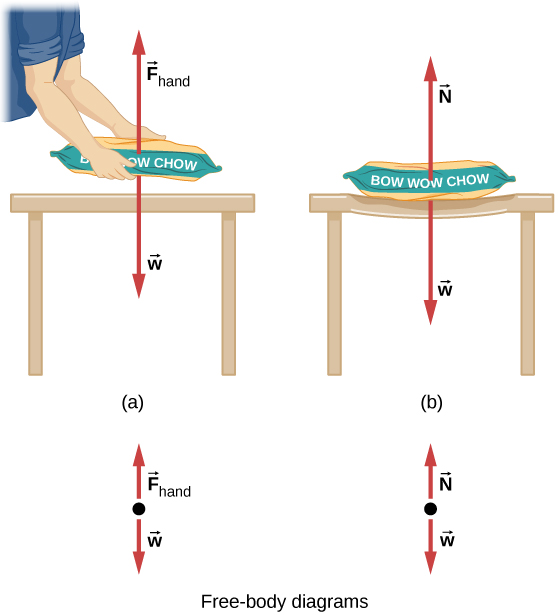Figure 5.21 (a) The person holding the bag of dog food must supply an upward force ${\overset{\to }{F}}_{\text{hand}}$ equal in magnitude and opposite in direction to the weight of the food $\overset{\to }{w}$ so that it doesn’t drop to the ground. (b) The card table sags when the dog food is placed on it, much like a stiff trampoline. Elastic restoring forces in the table grow as it sags until they supply a force $\overset{\to }{N}$ equal in magnitude and opposite in direction to the weight of the load.

We must conclude that whatever supports a load, be it animate or not, must supply an upward force equal to the weight of the load, as we assumed in a few of the previous examples. If the force supporting the weight of an object, or a load, is perpendicular to the surface of contact between the load and its support, this force is defined as a normal force and here is given by the symbol $\overset{\to }{N}.$ (This is not the newton unit for force, or N.) The word normal means perpendicular to a surface. This means that the normal force experienced by an object resting on a horizontal surface can be expressed in vector form as follows:

$\overset{\to }{N}=\text{−}m\overset{\to }{g}.$

In scalar form, this becomes

$N=mg.$

The normal force can be less than the object’s weight if the object is on an incline.

### Example

#### Weight on an Incline

Consider the skier on the slope in (Figure). Her mass including equipment is 60.0 kg. (a) What is her acceleration if friction is negligible? (b) What is her acceleration if friction is 45.0 N?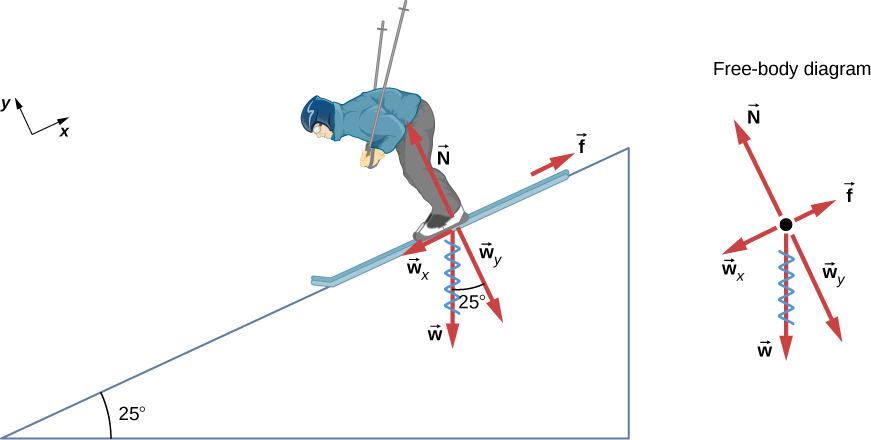Figure 5.22 Since the acceleration is parallel to the slope and acting down the slope, it is most convenient to project all forces onto a coordinate system where one axis is parallel to the slope and the other is perpendicular to it (axes shown to the left of the skier). $\overset{\to }{N}$ is perpendicular to the slope and $\overset{\to }{f}$ is parallel to the slope, but $\overset{\to }{w}$ has components along both axes, namely, ${w}_{y}$ and ${w}_{x}$. Here, $\overset{\to }{w}$ has a squiggly line to show that it has been replaced by these components. The force $\overset{\to }{N}$ is equal in magnitude to ${w}_{y}$, so there is no acceleration perpendicular to the slope, but f is less than ${w}_{x}$, so there is a downslope acceleration (along the axis parallel to the slope).

#### Strategy

This is a two-dimensional problem, since not all forces on the skier (the system of interest) are parallel. The approach we have used in two-dimensional kinematics also works well here. Choose a convenient coordinate system and project the vectors onto its axes, creating two one-dimensional problems to solve. The most convenient coordinate system for motion on an incline is one that has one coordinate parallel to the slope and one perpendicular to the slope. (Motions along mutually perpendicular axes are independent.) We use x and y for the parallel and perpendicular directions, respectively. This choice of axes simplifies this type of problem, because there is no motion perpendicular to the slope and the acceleration is downslope. Regarding the forces, friction is drawn in opposition to motion (friction always opposes forward motion) and is always parallel to the slope, ${w}_{x}$ is drawn parallel to the slope and downslope (it causes the motion of the skier down the slope), and ${w}_{y}$ is drawn as the component of weight perpendicular to the slope. Then, we can consider the separate problems of forces parallel to the slope and forces perpendicular to the slope.

#### Solution

The magnitude of the component of weight parallel to the slope is

${w}_{x}=w\,\text{sin}\,25\text{°}=mg\,\text{sin}\,25\text{°},$

and the magnitude of the component of the weight perpendicular to the slope is

${w}_{y}=w\,\text{cos}\,25\text{°}=mg\,\text{cos}\,25\text{°}.$

a. Neglect friction. Since the acceleration is parallel to the slope, we need only consider forces parallel to the slope. (Forces perpendicular to the slope add to zero, since there is no acceleration in that direction.) The forces parallel to the slope are the component of the skier’s weight parallel to slope ${w}_{x}$ and friction f. Using Newton’s second law, with subscripts to denote quantities parallel to the slope,

${a}_{x}=\frac{{F}_{\text{net}\,x}}{m}$

where ${F}_{\text{net}\,x}={w}_{x}-mg\,\text{sin}\,25\text{°},$ assuming no friction for this part. Therefore,

$\begin{array}{cc} {a}_{x}=\frac{{F}_{\text{net}\,x}}{m}=\frac{mg\,\text{sin}\,25\text{°}}{m}=g\,\text{sin}\,25\text{°}\hfill \\ (9.80\,{\text{m/s}}^{2})(0.4226)=4.14\,{\text{m/s}}^{2}\hfill \end{array}$

is the acceleration.

b. Include friction. We have a given value for friction, and we know its direction is parallel to the slope and it opposes motion between surfaces in contact. So the net external force is

${F}_{\text{net}\,x}={w}_{x}-f.$

Substituting this into Newton’s second law, ${a}_{x}={F}_{\text{net}\,x}\text{/}m,$ gives

${a}_{x}=\frac{{F}_{\text{net}\,x}}{m}=\frac{{w}_{x}-f}{m}=\frac{mg\,\text{sin}\,25\text{°}-f}{m}.$

We substitute known values to obtain

${a}_{x}=\frac{(60.0\,\text{kg})(9.80\,{\text{m/s}}^{2})(0.4226)-45.0\,\text{N}}{60.0\,\text{kg}}.$

This gives us

${a}_{x}=3.39\,{\text{m/s}}^{2},$

which is the acceleration parallel to the incline when there is 45.0 N of opposing friction.

#### Significance

Since friction always opposes motion between surfaces, the acceleration is smaller when there is friction than when there is none. It is a general result that if friction on an incline is negligible, then the acceleration down the incline is $a=g\,\text{sin}\,\theta$, regardless of mass. As discussed previously, all objects fall with the same acceleration in the absence of air resistance. Similarly, all objects, regardless of mass, slide down a frictionless incline with the same acceleration (if the angle is the same).

When an object rests on an incline that makes an angle $\theta$ with the horizontal, the force of gravity acting on the object is divided into two components: a force acting perpendicular to the plane, ${w}_{y}$, and a force acting parallel to the plane, ${w}_{x}$ ((Figure)). The normal force $\overset{\to }{N}$ is typically equal in magnitude and opposite in direction to the perpendicular component of the weight ${w}_{y}$. The force acting parallel to the plane, ${w}_{x}$, causes the object to accelerate down the incline.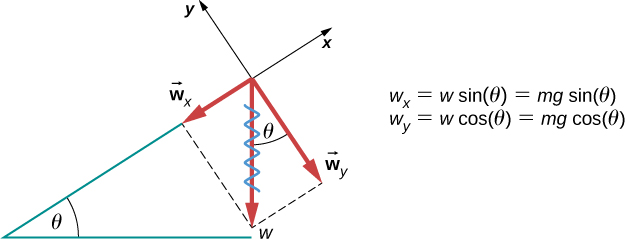Figure 5.23 An object rests on an incline that makes an angle $\theta$ with the horizontal.

Be careful when resolving the weight of the object into components. If the incline is at an angle $\theta$ to the horizontal, then the magnitudes of the weight components are

${w}_{x}=w\,\text{sin}\,\theta =mg\,\text{sin}\,\theta$

and

${w}_{y}=w\,\text{cos}\,\theta =mg\,\text{cos}\,\theta .$

We use the second equation to write the normal force experienced by an object resting on an inclined plane:

$N=mg\,\text{cos}\,\theta .$

Instead of memorizing these equations, it is helpful to be able to determine them from reason. To do this, we draw the right angle formed by the three weight vectors. The angle $\theta$ of the incline is the same as the angle formed between w and ${w}_{y}$. Knowing this property, we can use trigonometry to determine the magnitude of the weight components:

$\begin{array}{cc} \text{cos}\,\theta =\frac{{w}_{y}}{w},\enspace{w}_{y}=w\,\text{cos}\,\theta =mg\,\text{sin}\,\theta \hfill \\ \text{sin}\,\theta =\frac{{w}_{x}}{w},\enspace{w}_{x}=w\,\text{sin}\,\theta =mg\,\text{sin}\,\theta .\hfill \end{array}$

A force of 1150 N acts parallel to a ramp to push a 250-kg gun safe into a moving van. The ramp is frictionless and inclined at $17\text{°}.$ (a) What is the acceleration of the safe up the ramp? (b) If we consider friction in this problem, with a friction force of 120 N, what is the acceleration of the safe?

#### Tension

A tension is a force along the length of a medium; in particular, it is a pulling force that acts along a stretched flexible connector, such as a rope or cable. The word “tension” comes from a Latin word meaning “to stretch.” Not coincidentally, the flexible cords that carry muscle forces to other parts of the body are called tendons.

Any flexible connector, such as a string, rope, chain, wire, or cable, can only exert a pull parallel to its length; thus, a force carried by a flexible connector is a tension with a direction parallel to the connector. Tension is a pull in a connector. Consider the phrase: “You can’t push a rope.” Instead, tension force pulls outward along the two ends of a rope.

Consider a person holding a mass on a rope, as shown in (Figure). If the 5.00-kg mass in the figure is stationary, then its acceleration is zero and the net force is zero. The only external forces acting on the mass are its weight and the tension supplied by the rope. Thus,

${F}_{\text{net}}=T-w=0,$

where T and w are the magnitudes of the tension and weight, respectively, and their signs indicate direction, with up being positive. As we proved using Newton’s second law, the tension equals the weight of the supported mass:

$T=w=mg.$

Thus, for a 5.00-kg mass (neglecting the mass of the rope), we see that

$T=mg=(5.00\,\text{kg})(9.80\,{\text{m/s}}^{2})=49.0\,\text{N}\text{.}$

If we cut the rope and insert a spring, the spring would extend a length corresponding to a force of 49.0 N, providing a direct observation and measure of the tension force in the rope.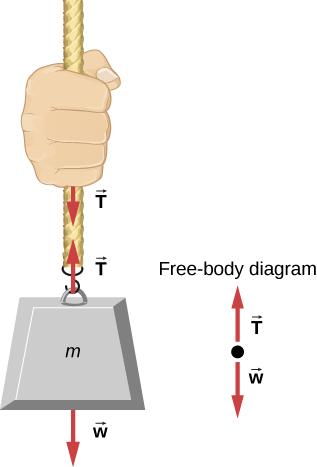Figure 5.24 When a perfectly flexible connector (one requiring no force to bend it) such as this rope transmits a force $\overset{\to }{T},$ that force must be parallel to the length of the rope, as shown. By Newton’s third law, the rope pulls with equal force but in opposite directions on the hand and the supported mass (neglecting the weight of the rope). The rope is the medium that carries the equal and opposite forces between the two objects. The tension anywhere in the rope between the hand and the mass is equal. Once you have determined the tension in one location, you have determined the tension at all locations along the rope.

Flexible connectors are often used to transmit forces around corners, such as in a hospital traction system, a tendon, or a bicycle brake cable. If there is no friction, the tension transmission is undiminished; only its direction changes, and it is always parallel to the flexible connector, as shown in (Figure).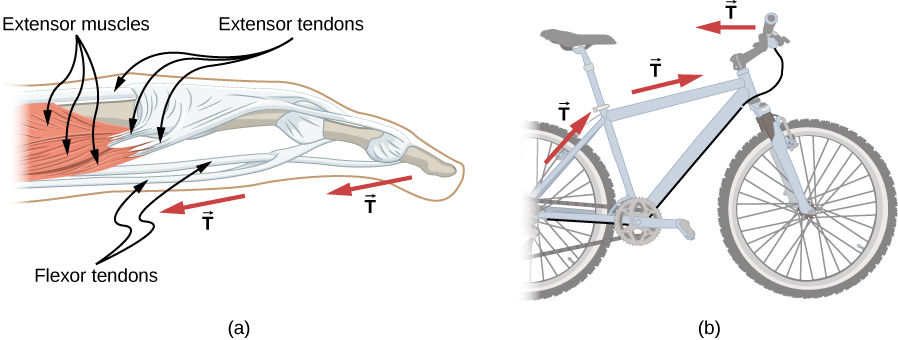Figure 5.25 (a) Tendons in the finger carry force T from the muscles to other parts of the finger, usually changing the force’s direction but not its magnitude (the tendons are relatively friction free). (b) The brake cable on a bicycle carries the tension T from the brake lever on the handlebars to the brake mechanism. Again, the direction but not the magnitude of T is changed.

### Example

#### What Is the Tension in a Tightrope?

Calculate the tension in the wire supporting the 70.0-kg tightrope walker shown in (Figure).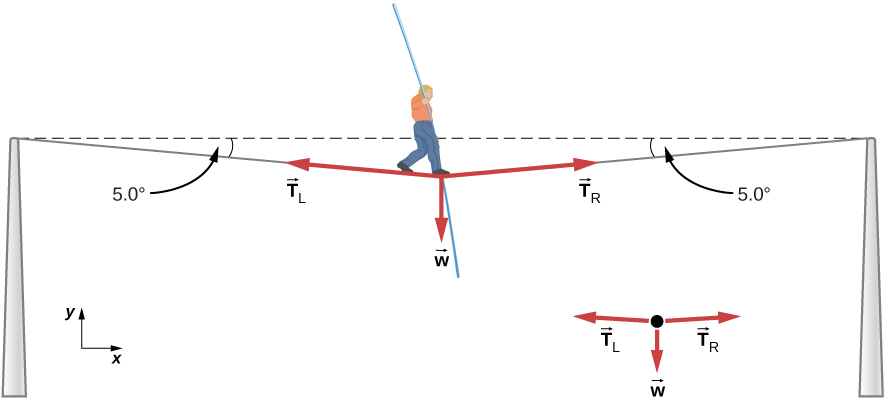Figure 5.26 The weight of a tightrope walker causes a wire to sag by $5.0\text{°}$. The system of interest is the point in the wire at which the tightrope walker is standing.

#### Strategy

As you can see in (Figure), the wire is bent under the person’s weight. Thus, the tension on either side of the person has an upward component that can support his weight. As usual, forces are vectors represented pictorially by arrows that have the same direction as the forces and lengths proportional to their magnitudes. The system is the tightrope walker, and the only external forces acting on him are his weight $\overset{\to }{w}$ and the two tensions ${\overset{\to }{T}}_{\text{L}}$ (left tension) and ${\overset{\to }{T}}_{\text{R}}$ (right tension). It is reasonable to neglect the weight of the wire. The net external force is zero, because the system is static. We can use trigonometry to find the tensions. One conclusion is possible at the outset—we can see from (Figure)(b) that the magnitudes of the tensions ${T}_{\text{L}}$ and ${T}_{\text{R}}$ must be equal. We know this because there is no horizontal acceleration in the rope and the only forces acting to the left and right are ${T}_{\text{L}}$ and ${T}_{\text{R}}$. Thus, the magnitude of those horizontal components of the forces must be equal so that they cancel each other out.

Whenever we have two-dimensional vector problems in which no two vectors are parallel, the easiest method of solution is to pick a convenient coordinate system and project the vectors onto its axes. In this case, the best coordinate system has one horizontal axis (x) and one vertical axis (y).

#### Solution

First, we need to resolve the tension vectors into their horizontal and vertical components. It helps to look at a new free-body diagram showing all horizontal and vertical components of each force acting on the system ((Figure)).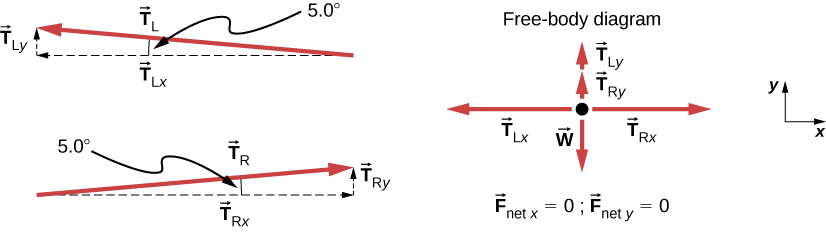Figure 5.27 When the vectors are projected onto vertical and horizontal axes, their components along these axes must add to zero, since the tightrope walker is stationary. The small angle results in T being much greater than w.

Consider the horizontal components of the forces (denoted with a subscript x):

${F}_{\text{net}\,x}={T}_{\text{R}x}-{T}_{\text{L}x}.$

The net external horizontal force ${F}_{\text{net}\,x}=0,$ since the person is stationary. Thus,

$\begin{array}{ccc}\hfill {F}_{\text{net}\,x}& =\hfill & 0={T}_{\text{R}x}-{T}_{\text{L}x}\hfill \\ \hfill {T}_{\text{L}x}& =\hfill & {T}_{\text{R}x}.\hfill \end{array}$

Now observe (Figure). You can use trigonometry to determine the magnitude of ${T}_{\text{L}}$ and ${T}_{\text{R}}$:

$\begin{array}{cc} \hfill \text{cos}\,5.0\text{°}& =\hfill & \frac{{T}_{\text{L}x}}{{T}_{\text{L}}},\enspace{T}_{\text{L}x}={T}_{\text{L}}\text{cos}\,5.0\text{°}\hfill \\ \hfill \text{cos}\,5.0\text{°}& =\hfill & \frac{{T}_{\text{R}x}}{{T}_{\text{R}}},\enspace{T}_{\text{R}x}={T}_{\text{R}}\text{cos}\,5.0\text{°}.\hfill \end{array}$

Equating TLx and TRx:

${T}_{\text{L}}\text{cos}\,5.0\text{°}={T}_{\text{R}}\text{cos}\,5.0\text{°}.$

Thus,

${T}_{\text{L}}={T}_{\text{R}}=T,$

as predicted. Now, considering the vertical components (denoted by a subscript y), we can solve for T. Again, since the person is stationary, Newton’s second law implies that ${F}_{\text{net}\,y}=0$. Thus, as illustrated in the free-body diagram,

${F}_{\text{net}\,y}={T}_{\text{L}y}+{T}_{\text{R}y}-w=0.$

We can use trigonometry to determine the relationships among ${T}_{\text{Ly}},{T}_{\text{Ry}},$ and T. As we determined from the analysis in the horizontal direction, ${T}_{\text{L}}={T}_{\text{R}}=T$:

$\begin{array}{}\\ \hfill \text{sin}\,5.0\text{°}& =\hfill & \frac{{T}_{\text{L}y}}{{T}_{\text{L}}},\enspace{T}_{\text{L}y}={T}_{\text{L}}\text{sin}\,5.0\text{°}=T\,\text{sin}\,5.0\text{°}\hfill \\ \hfill \text{sin}\,5.0\text{°}& =\hfill & \frac{{T}_{\text{R}y}}{{T}_{\text{R}}},\enspace{T}_{\text{R}y}={T}_{\text{R}}\text{sin}\,5.0\text{°}=T\,\text{sin}\,5.0\text{°}.\hfill \end{array}$

Now we can substitute the vales for ${T}_{\text{Ly}}$ and ${T}_{\text{Ry}}$, into the net force equation in the vertical direction:

$\begin{array}{ccc}\hfill {F}_{\text{net}\,y}& =\hfill & {T}_{\text{L}y}+{T}_{\text{R}y}-w=0\hfill \\ \hfill {F}_{\text{net}\,y}& =\hfill & T\,\text{sin}\,5.0\text{°}+T\,\text{sin}\,5.0\text{°}-w=0\hfill \\ \hfill 2T\,\text{sin}\,5.0\text{°}-w& =\hfill & 0\hfill \\ \hfill 2T\,\text{sin}\,5.0\text{°}& =\hfill & w\hfill \end{array}$

and

$T=\frac{w}{2\,\text{sin}\,5.0\text{°}}=\frac{mg}{2\,\text{sin}\,5.0\text{°}},$

so

$T=\frac{(70.0\,\text{kg})(9.80\,{\text{m/s}}^{2})}{2(0.0872)},$

and the tension is

$T=3930\,\text{N}\text{.}$

#### Significance

The vertical tension in the wire acts as a force that supports the weight of the tightrope walker. The tension is almost six times the 686-N weight of the tightrope walker. Since the wire is nearly horizontal, the vertical component of its tension is only a fraction of the tension in the wire. The large horizontal components are in opposite directions and cancel, so most of the tension in the wire is not used to support the weight of the tightrope walker.

If we wish to create a large tension, all we have to do is exert a force perpendicular to a taut flexible connector, as illustrated in (Figure). As we saw in (Figure), the weight of the tightrope walker acts as a force perpendicular to the rope. We saw that the tension in the rope is related to the weight of the tightrope walker in the following way:

$T=\frac{w}{2\,\text{sin}\,\theta }.$

We can extend this expression to describe the tension T created when a perpendicular force $({F}_{\perp })$ is exerted at the middle of a flexible connector:

$T=\frac{{F}_{\perp }}{2\,\text{sin}\,\theta }.$

The angle between the horizontal and the bent connector is represented by $\theta$. In this case, T becomes large as $\theta$ approaches zero. Even the relatively small weight of any flexible connector will cause it to sag, since an infinite tension would result if it were horizontal (i.e., $\theta =0$ and sin $\theta =0$). For example, (Figure) shows a situation where we wish to pull a car out of the mud when no tow truck is available. Each time the car moves forward, the chain is tightened to keep it as straight as possible. The tension in the chain is given by $T=\frac{{F}_{\perp }}{2\,\text{sin}\,\theta },$ and since $\theta$ is small, T is large. This situation is analogous to the tightrope walker, except that the tensions shown here are those transmitted to the car and the tree rather than those acting at the point where ${F}_{\perp }$ is applied.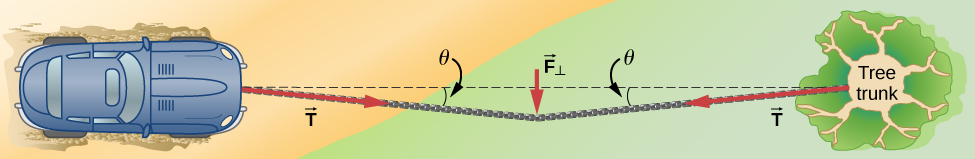Figure 5.28 We can create a large tension in the chain—and potentially a big mess—by pushing on it perpendicular to its length, as shown.

One end of a 3.0-m rope is tied to a tree; the other end is tied to a car stuck in the mud. The motorist pulls sideways on the midpoint of the rope, displacing it a distance of 0.25 m. If he exerts a force of 200.0 N under these conditions, determine the force exerted on the car.

In Applications of Newton’s Laws, we extend the discussion on tension in a cable to include cases in which the angles shown are not equal.

#### Friction

Friction is a resistive force opposing motion or its tendency. Imagine an object at rest on a horizontal surface. The net force acting on the object must be zero, leading to equality of the weight and the normal force, which act in opposite directions. If the surface is tilted, the normal force balances the component of the weight perpendicular to the surface. If the object does not slide downward, the component of the weight parallel to the inclined plane is balanced by friction. Friction is discussed in greater detail in the next chapter.

#### Spring force

A spring is a special medium with a specific atomic structure that has the ability to restore its shape, if deformed. To restore its shape, a spring exerts a restoring force that is proportional to and in the opposite direction in which it is stretched or compressed. This is the statement of a law known as Hooke’s law, which has the mathematical form

$\overset{\to }{F}=\text{−}k\overset{\to }{x}.$

The constant of proportionality k is a measure of the spring’s stiffness. The line of action of this force is parallel to the spring axis, and the sense of the force is in the opposite direction of the displacement vector ((Figure)). The displacement must be measured from the relaxed position; $x=0$ when the spring is relaxed.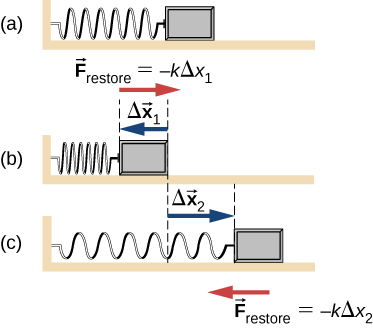Figure 5.29 A spring exerts its force proportional to a displacement, whether it is compressed or stretched. (a) The spring is in a relaxed position and exerts no force on the block. (b) The spring is compressed by displacement $\text{Δ}{\overset{\to }{x}}_{1}$ of the object and exerts restoring force $\text{−}k\text{Δ}{\overset{\to }{x}}_{1}.$ (c) The spring is stretched by displacement $\text{Δ}{\overset{\to }{x}}_{2}$ of the object and exerts restoring force $\text{−}k\text{Δ}{\overset{\to }{x}}_{2}.$

### Real Forces and Inertial Frames

There is another distinction among forces: Some forces are real, whereas others are not. Real forces have some physical origin, such as a gravitational pull. In contrast, fictitious forces arise simply because an observer is in an accelerating or noninertial frame of reference, such as one that rotates (like a merry-go-round) or undergoes linear acceleration (like a car slowing down). For example, if a satellite is heading due north above Earth’s Northern Hemisphere, then to an observer on Earth, it will appear to experience a force to the west that has no physical origin. Instead, Earth is rotating toward the east and moves east under the satellite. In Earth’s frame, this looks like a westward force on the satellite, or it can be interpreted as a violation of Newton’s first law (the law of inertia). We can identify a fictitious force by asking the question, “What is the reaction force?” If we cannot name the reaction force, then the force we are considering is fictitious. In the example of the satellite, the reaction force would have to be an eastward force on Earth. Recall that an inertial frame of reference is one in which all forces are real and, equivalently, one in which Newton’s laws have the simple forms given in this chapter.

Earth’s rotation is slow enough that Earth is nearly an inertial frame. You ordinarily must perform precise experiments to observe fictitious forces and the slight departures from Newton’s laws, such as the effect just described. On a large scale, such as for the rotation of weather systems and ocean currents, the effects can be easily observed ((Figure)).Figure 5.30 Hurricane Fran is shown heading toward the southeastern coast of the United States in September 1996. Notice the characteristic “eye” shape of the hurricane. This is a result of the Coriolis effect, which is the deflection of objects (in this case, air) when considered in a rotating frame of reference, like the spin of Earth.

The crucial factor in determining whether a frame of reference is inertial is whether it accelerates or rotates relative to a known inertial frame. Unless stated otherwise, all phenomena discussed in this text are in inertial frames.

The forces discussed in this section are real forces, but they are not the only real forces. Lift and thrust, for example, are more specialized real forces. In the long list of forces, are some more basic than others? Are some different manifestations of the same underlying force? The answer to both questions is yes, as you will see in the treatment of modern physics later in the text.

Explore forces and motion in this interactive simulation as you push household objects up and down a ramp. Lower and raise the ramp to see how the angle of inclination affects the parallel forces. Graphs show forces, energy, and work.

Stretch and compress springs in this activity to explore the relationships among force, spring constant, and displacement. Investigate what happens when two springs are connected in series and in parallel.

### Summary

• When an object rests on a surface, the surface applies a force to the object that supports the weight of the object. This supporting force acts perpendicular to and away from the surface. It is called a normal force.
• When an object rests on a nonaccelerating horizontal surface, the magnitude of the normal force is equal to the weight of the object.
• When an object rests on an inclined plane that makes an angle $\theta$ with the horizontal surface, the weight of the object can be resolved into components that act perpendicular and parallel to the surface of the plane.
• The pulling force that acts along a stretched flexible connector, such as a rope or cable, is called tension. When a rope supports the weight of an object at rest, the tension in the rope is equal to the weight of the object. If the object is accelerating, tension is greater than weight, and if it is decelerating, tension is less than weight.
• The force of friction is a force experienced by a moving object (or an object that has a tendency to move) parallel to the interface opposing the motion (or its tendency).
• The force developed in a spring obeys Hooke’s law, according to which its magnitude is proportional to the displacement and has a sense in the opposite direction of the displacement.
• Real forces have a physical origin, whereas fictitious forces occur because the observer is in an accelerating or noninertial frame of reference.

### Conceptual Questions

A table is placed on a rug. Then a book is placed on the table. What does the floor exert a normal force on?

A particle is moving to the right. (a) Can the force on it to be acting to the left? If yes, what would happen? (b) Can that force be acting downward? If yes, why?

### Problems

A leg is suspended in a traction system, as shown below. (a) Which pulley in the figure is used to calculate the force exerted on the foot? (b) What is the tension in the rope? Here $\overset{\to }{T}$ is the tension, ${\overset{\to }{w}}_{\text{leg}}$ is the weight of the leg, and $\overset{\to }{w}$ is the weight of the load that provides the tension.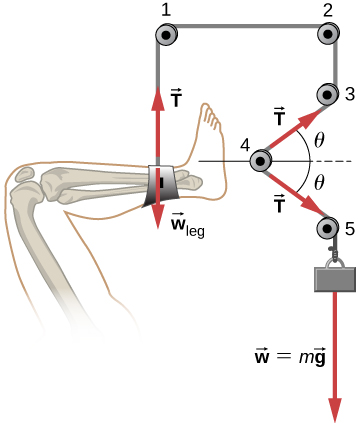Suppose the shinbone in the preceding image was a femur in a traction setup for a broken bone, with pulleys and rope available. How might we be able to increase the force along the femur using the same weight?

Two teams of nine members each engage in tug-of-war. Each of the first team’s members has an average mass of 68 kg and exerts an average force of 1350 N horizontally. Each of the second team’s members has an average mass of 73 kg and exerts an average force of 1365 N horizontally. (a) What is magnitude of the acceleration of the two teams, and which team wins? (b) What is the tension in the section of rope between the teams?

What force does a trampoline have to apply to Jennifer, a 45.0-kg gymnast, to accelerate her straight up at $7.50\,{\text{m/s}}^{2}$? The answer is independent of the velocity of the gymnast—she can be moving up or down or can be instantly stationary.

(a) Calculate the tension in a vertical strand of spider web if a spider of mass $2.00\,×\,{10}^{-5}\,\text{kg}$ hangs motionless on it. (b) Calculate the tension in a horizontal strand of spider web if the same spider sits motionless in the middle of it much like the tightrope walker in (Figure). The strand sags at an angle of $12\text{°}$ below the horizontal. Compare this with the tension in the vertical strand (find their ratio).

Suppose Kevin, a 60.0-kg gymnast, climbs a rope. (a) What is the tension in the rope if he climbs at a constant speed? (b) What is the tension in the rope if he accelerates upward at a rate of $1.50\,{\text{m/s}}^{2}$?

Show that, as explained in the text, a force ${F}_{\perp }$ exerted on a flexible medium at its center and perpendicular to its length (such as on the tightrope wire in (Figure)) gives rise to a tension of magnitude $T={F}_{\perp }\text{/}2\,\text{sin}(\theta )$.

Consider (Figure). The driver attempts to get the car out of the mud by exerting a perpendicular force of 610.0 N, and the distance she pushes in the middle of the rope is 1.00 m while she stands 6.00 m away from the car on the left and 6.00 m away from the tree on the right. What is the tension T in the rope, and how do you find the answer?

A bird has a mass of 26 g and perches in the middle of a stretched telephone line. (a) Show that the tension in the line can be calculated using the equation $T=\frac{mg}{2\,\text{sin}\,\theta }$. Determine the tension when (b) $\theta =5\text{°}$ and (c) $\theta =0.5\text{°}$. Assume that each half of the line is straight.One end of a 30-m rope is tied to a tree; the other end is tied to a car stuck in the mud. The motorist pulls sideways on the midpoint of the rope, displacing it a distance of 2 m. If he exerts a force of 80 N under these conditions, determine the force exerted on the car.

Consider the baby being weighed in the following figure. (a) What is the mass of the infant and basket if a scale reading of 55 N is observed? (b) What is tension ${T}_{1}$ in the cord attaching the baby to the scale? (c) What is tension ${T}_{2}$ in the cord attaching the scale to the ceiling, if the scale has a mass of 0.500 kg? (d) Sketch the situation, indicating the system of interest used to solve each part. The masses of the cords are negligible.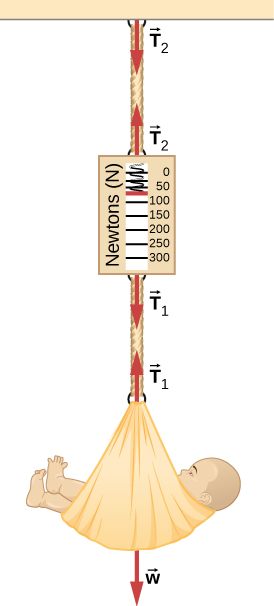What force must be applied to a 100.0-kg crate on a frictionless plane inclined at $30\text{°}$ to cause an acceleration of ${2.0\,\text{m/s}}^{2}$ up the plane?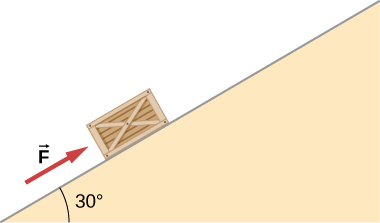A 2.0-kg block is on a perfectly smooth ramp that makes an angle of $30\text{°}$ with the horizontal. (a) What is the block’s acceleration down the ramp and the force of the ramp on the block? (b) What force applied upward along and parallel to the ramp would allow the block to move with constant velocity?

### Glossary

Hooke’s law
in a spring, a restoring force proportional to and in the opposite direction of the imposed displacement
normal force
force supporting the weight of an object, or a load, that is perpendicular to the surface of contact between the load and its support; the surface applies this force to an object to support the weight of the object
tension
pulling force that acts along a stretched flexible connector, such as a rope or cable